基于MATLAB的视频运动目标检测识别

100帧视频图像的读取

if nargin==0

i=1;min=00000001;

end

name=num2str(i);

if i<=9

min=strcat('0000000',name,'.bmp');

elseif i<=99

min=strcat('000000',name,'.bmp');

else

min=strcat('00000',name,'.bmp');

end

(1)简单阈值分割法;

(2)多阈值分割法;

(3)最大类间方差法;

(4)最佳阈值法。(5-1)

figure(1),imshow(I0);%原图像显示

I0=double(I0);%化为双精度

figure(2),hist(I0,300);%原图像直方图显示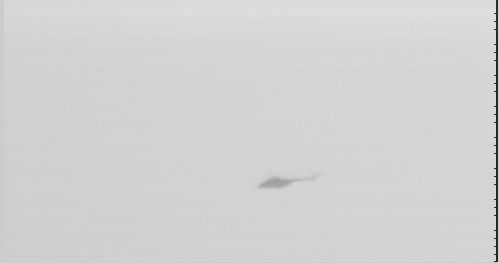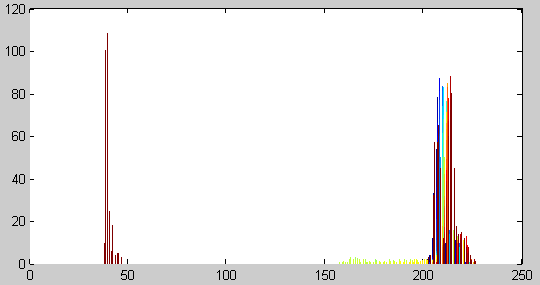T1=50;T2=200;%观察并找出阈值

for i=1:200

M=double(I);

for m=1:272

for n=1:512

if (M(m,n)>=T1)&&(M(m,n)<=T2)

M(m,n)=1;%设置背景灰度

else

M(m,n)=0;%设置目标灰度

end

end

end%%图像的分割和阈值处理

end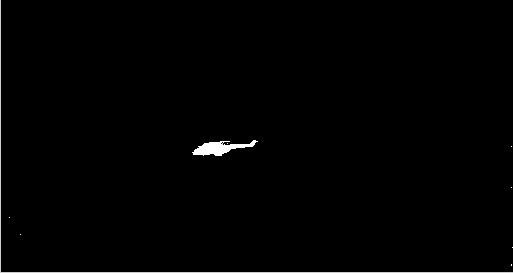（5-2）

for i=1:200

X=0;Y=0;X1=0;Y1=0;PINJUN=0;PINJUN1=0;

for m=1:272

for n=1:512

x=m*M(m,n); y=n*M(m,n); pinjun=M(m,n);

X=X+x;Y=Y+y;PINJUN=PINJUN+pinjun;

end

X1=X1+X;

Y1=Y1+Y;

PINJUN1=PINJUN1+PINJUN;

end

Xmean=X1/PINJUN1;

Ymean=Y1/PINJUN1; %求取形心的计算

Xmean=(Xmean*100-mod(Xmean*100,100))/100;

Ymean=(Ymean*100-mod(Ymean*100,100))/100;%形心数值取整

end

for m=(Xmean-16):(Xmean+16)

n=Ymean-32;

I(m,n)=1;

end

for m=(Xmean-16):(Xmean+16)

n=Ymean+32;

I(m,n)=1;

end

for n=(Ymean-32):(Ymean+32)

m=Xmean-16;

I(m,n)=1;

end

for n=(Ymean-32):(Ymean+32)

m=Xmean+16;

I(m,n)=1;

end%画出跟踪波门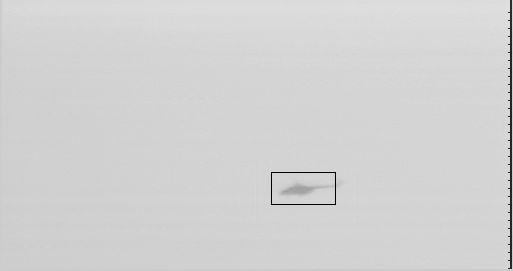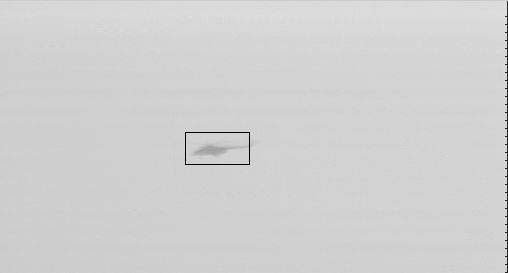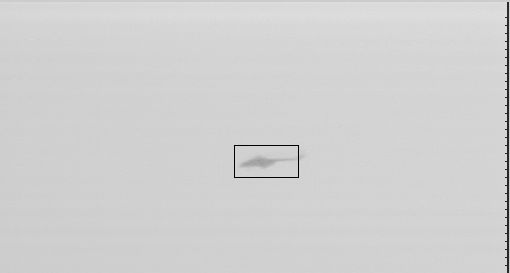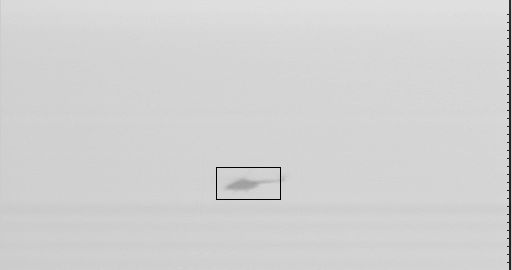figure(1),imshow(I0);%原图像显示

I0=double(I0);

figure(2),hist(I0,300);%原图像直方图显示

T1=50;T2=200;%观察并找出阈值

for i=1:200

M=double(I);

for m=1:272

for n=1:512

if (M(m,n)>=T1)&&(M(m,n)<=T2)

M(m,n)=1;%设置背景灰度

else

M(m,n)=0;%设置目标灰度

end

end

end%%图像的分割和阈值处理

X=0;Y=0;X1=0;Y1=0;PINJUN=0;PINJUN1=0;

for m=1:272

for n=1:512

x=m*M(m,n); y=n*M(m,n); pinjun=M(m,n);

X=X+x;Y=Y+y;PINJUN=PINJUN+pinjun;

end

X1=X1+X;

Y1=Y1+Y;

PINJUN1=PINJUN1+PINJUN;

end

Xmean=X1/PINJUN1;

Ymean=Y1/PINJUN1;

%求取形心的计算

for m=(Xmean-16):(Xmean+16)

n=Ymean-32;

I(m,n)=1;

end

for m=(Xmean-16):(Xmean+16)

n=Ymean+32;

I(m,n)=1;

end

for n=(Ymean-32):(Ymean+32)

m=Xmean-16;

I(m,n)=1;

end

for n=(Ymean-32):(Ymean+32)

m=Xmean+16;

I(m,n)=1;

end%画出跟踪波门

figure(3),imshow(I);%连续读出每一帧图像，连续显示已达到视频播放的效果。

12-03
10-2403-27
08-14
11-09
05-316258
08-22341
08-22738
12-319834
06-27
12-088254
03-20525
03-16145
12-037251sunny_chenxi

¥2 ¥4 ¥6 ¥10 ¥20余额支付 (余额：-- )扫码支付获取中扫码支付点击重新获取扫码支付1.余额是钱包充值的虚拟货币，按照1:1的比例进行支付金额的抵扣。
2.余额无法直接购买下载，可以购买VIP、C币套餐、付费专栏及课程。余额充值